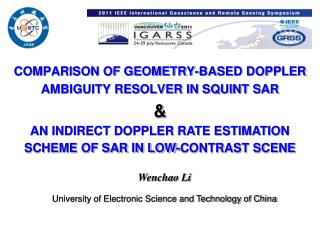DownloadDownload PresentationCOMPARISON OF GEOMETRY-BASED DOPPLER AMBIGUITY RESOLVER IN SQUINT SAR &

# COMPARISON OF GEOMETRY-BASED DOPPLER AMBIGUITY RESOLVER IN SQUINT SAR &

Télécharger la présentation## COMPARISON OF GEOMETRY-BASED DOPPLER AMBIGUITY RESOLVER IN SQUINT SAR &

- - - - - - - - - - - - - - - - - - - - - - - - - - - E N D - - - - - - - - - - - - - - - - - - - - - - - - - - -
##### Presentation Transcript

1. COMPARISON OF GEOMETRY-BASED DOPPLER AMBIGUITY RESOLVER IN SQUINT SAR& AN INDIRECT DOPPLER RATE ESTIMATION SCHEME OF SAR IN LOW-CONTRAST SCENE Wenchao Li University of Electronic Science and Technology of China

2. Introduction Synthetic Aperture Radar (SAR) An important remote sensing tool. • Monostatic SAR • Bistatic SAR • Stripmap SAR • Scan SAR • Spotlight SAR • Side-looking SAR • Squint-looking SAR • ……

3. Introduction Squint SAR Squint angle Geometry model

4. Introduction Squint SAR Range History： • The linear term is range walk • The quadratic term is range curvature

5. Introduction Signal model After range walk correction, the azimuth data can be modeled as a linear frequency modulated (LFM) signal, • Doppler centroid ( ) • Doppler rate ( )

6. Introduction In principle, it is possible to compute Doppler parameter from prior information provided by GPS/INS, but measurement uncertainties on the information will limit the accuracy.

7. Introduction • An incorrect Doppler centroid • a loss of SNR; • an increase in the azimuth ambiguity level; • a shift in the location of the target; • …... An incorrect Doppler rate would blur the image. Correct Doppler rate Incorrect Doppler rate

8. Introduction Therefore, Doppler parameter Estimation from echo data is an essential procedure for high quality SAR processing

9. Doppler centroid estimation (Resolve Doppler centroid ambiguity)

10. Doppler centroid estimation As azimuth data are sampled by pulse repetition frequency (PRF), there is always Doppler centroid ambiguity, and the bigger the squint angle is, the more serious the doppler ambiguity will be. Doppler centroid is usually expressed in two parts: the baseband Doppler centroid and Doppler ambiguity number .

11. Doppler centroid estimation • Multiple PRF • Wavelength diversity algorithm (WDA) • Multilook algorithms (MLCC,MLBF) • Geometry-based algorithms

12. Geometry-based algorithms The relationship between Doppler centroid and range walk slope, Then Doppler centroid can be computed via estimating the range walk slope. Since this method is based on the geometric characteristics of the range compressed data, it is named as a geometry-based Doppler ambiguity resolver.

13. Geometry-based algorithms Conventional Radon transform scheme • Radon transform: • A well-known tool for detecting slope. • It integrates intensity along every possible direction in the image and maps this information into a feature space. • It involves two-dimensional search.

14. Geometry-based algorithms Iterative scheme The slope is seen as a searching variable, and entropy is used to measure the robustness of signal. It utilizes the prior information of platform, and replaces the Radon transform with a simple integration of image intensity over azimuth.

15. Geometry-based algorithms Improved Radon transform scheme This scheme utilizes prior information of platform, convert the data into binary data, and conduct two-step Radon transform, which would make it is sparse and reduce the need to search the whole feature space .

16. Comparisons It is obviously that they can give the accurate Doppler ambiguity number. While the speed of the iterative scheme and the improved Radon transform scheme is much faster than conventional Radon transform.

17. Doppler rate estimation (Autofocus)

18. Doppler rate estimation Parametric Method • Metric-based Autofocus (Entropy/Contrast) • Map Drift (MD) • Shift and correlate (SAC) High contrast scene. Nonparametric Method • Prominent Point Processing (PPP) • Phase Gradient Autofocus (PGA) • Time-Frequency Analysis

19. Doppler rate estimation • Low contrast scene • Low SNR • Without prominent point • What can we do ?

20. Estimation Scheme According to the fact that Doppler rate is inversely proportional to range, It indicates that the Doppler rate in low- contrast scene can be estimated indirectly.

21. Estimation Flowchart Doppler rate in high-contrast scene is estimated first using Radon-Wigner transform, and then using the fact that Doppler rate is inversely proportional to range, Doppler rate in low-contrast scene is estimated indirectly.

22. Results High Contrast Scene With Prominent Point Time frequency analysis Radon transform Low Contrast Scene Without Prominent Point Time frequency analysis Radon transform

23. Conclusions • The geometry-based scheme can be used to resolve Doppler ambiguity of SAR with large migration. • The speed of improved Radon transform scheme and the iterative scheme is about ten times faster than conventional Radon transform scheme. • An indirect estimation scheme of Doppler rate for low contrast scene is developed. • The indirect scheme is effective, and it can reduce computation time greatly.

24. Thank you for your listening!